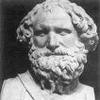You may also likeArchimedes and Numerical Roots

The problem is how did Archimedes calculate the lengths of the sides of the polygons which needed him to be able to calculate square roots?Time to Evolve

How many generations would link an evolutionist to a very distant ancestor?Where Am I?

From the information you are asked to work out where the picture was taken. Is there too much information? How accurate can your answer be?

More or Less?

Age 14 to 16 Challenge Level:

In each question there will be some way of relating the quantities referred to. You will need to think what equations from science to use.

Once you have the equations, what quantities don't you know? Could you substitute sensible values for one of these?

Don't forget to use physical intuition. For example, an apple floats in water. What does that tell you about its density?